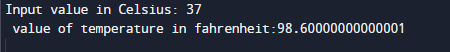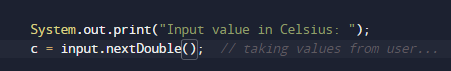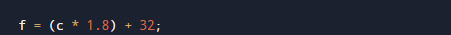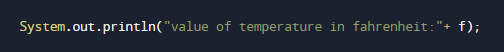# Java Program to Convert Celsius to Fahrenheit

In this tutorial you will learn about the Java Program to Convert Celsius to Fahrenheit and its application with practical example.

In this tutorial, we will learn to create a Java program that will Convert Celsius to Fahrenheit   using Java programming.

## Prerequisites

Before starting with this tutorial we assume that you are best aware of the following Java programming topics:

• Java Operators.
• Basic Input and Output
• Class and Object.
• Basic Java programming.

## What Is Temperature ?

Temperature is a quantity that measured the freezing and boiling point of Water. Which is measured in different scales in different regions like Celsius in India and Fahrenheit in European Countries.
In Celsius scale, the boiling point of water is 100°C, and the freezing point is at 0°C, while in the Fahrenheit scale the boiling point of water is 212°F and freezing point is at 32°F.

To convert values from Celsius  to Fahrenheit  we will use the following Formula:
f = (c * 9 / 5) + 32 where, F is temperature in Fahrenheit and C is temperature in Celsius.

## Java Program to Convert Celsius to Fahrenheit

In this program we will convert temperature from Celsius to Fahrenheit . We would first declared and initialized the required variables. Next, we would prompt user to input the value in Celsius. Later we will Convert the values into Fahrenheit.

## OutputConcept to find Centigrade to Fahrenheit.

First of all we will take the value Celsius Temperature in c.
Then using formula we calculate f = (c * 9 / 5) + 32.
Print  Fahrenheit.

In the above program, we have first declared and initialized a set variables required in the program.

• f = it will hold Fahrenheit value.
• c= it will hold Celsius value.

And  in the next statement user will be prompted to enter value in Celsius  and  which will be assigned to variable ‘c‘.After that with the help of  formula we will convert the value in to Fahrenheit .As you can see in our program, we first take value in Celsius  from user as input. Then convert the value of Celsius into Fahrenheit  using above conversion equation and print the Temperature in Fahrenheit .In this tutorial we have learn about the Java Program to Convert Celsius to Fahrenheit and its application with practical example. I hope you will like this tutorial.